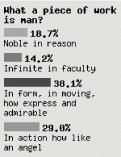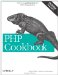# Recipe 17.10. Program: Generating Bar Charts from Poll Results

When displaying the results of a poll, it can be more effective to generate a colorful bar chart instead of just printing the results as text. The function shown in Example 17-4 uses GD to create an image that displays the cumulative responses to a poll question.

##### Graphical bar charts

 `function pc_bar_chart(\$question, \$answers) { // define colors to draw the bars \$colors = array(array(255,102,0), array(0,153,0), array(51,51,204), array(255,0,51), array(255,255,0), array(102,255,255), array(153,0,204)); \$total = array_sum(\$answers['votes']); // define some spacing values and other magic numbers \$padding = 5; \$line_width = 20; \$scale = \$line_width * 7.5; \$bar_height = 10; \$x = \$y = \$padding; // allocate a large palette for drawing, since you don't know // the image length ahead of time \$image = ImageCreate(150, 500); \$bg_color = ImageColorAllocate(\$image, 224, 224, 224); \$black = ImageColorAllocate(\$image, 0, 0, 0); // print the question \$wrapped = explode("\n", wordwrap(\$question, \$line_width)); foreach (\$wrapped as \$line) { ImageString(\$image, 3, \$x, \$y , \$line, \$black); \$y += 12; } \$y += \$padding; // print the answers for (\$i = 0; \$i < count(\$answers['answer']); \$i++) { // format percentage \$percent = sprintf('%1.1f', 100*\$answers['votes'][\$i]/\$total); \$bar = sprintf('%d', \$scale*\$answers['votes'][\$i]/\$total); // grab color \$c = \$i % count(\$colors); // handle cases with more bars than colors \$text_color = ImageColorAllocate(\$image, \$colors[\$c], \$colors[\$c], \$colors[\$c]); // draw bar and percentage numbers ImageFilledRectangle(\$image, \$x, \$y, \$x + \$bar, \$y + \$bar_height, \$text_color); ImageString(\$image, 3, \$x + \$bar + \$padding, \$y, "\$percent%", \$black); \$y += 12; // print answer \$wrapped = explode("\n", wordwrap(\$answers['answer'][\$i], \$line_width)); foreach (\$wrapped as \$line) { ImageString(\$image, 2, \$x, \$y, \$line, \$black); \$y += 12; } \$y += 7; } // crop image by copying it \$chart = ImageCreate(150, \$y); ImageCopy(\$chart, \$image, 0, 0, 0, 0, 150, \$y); // deliver image header ('Content-type: image/png'); ImagePNG(\$chart); // clean up ImageDestroy(\$image); ImageDestroy(\$chart); }`

`// Act II. Scene II. \$question = 'What a piece of work is man?'; \$answers['answer'][] = 'Noble in reason'; \$answers['votes'][]  = 29; \$answers['answer'][] = 'Infinite in faculty'; \$answers['votes'][]  = 22; \$answers['answer'][] = 'In form, in moving, how express and admirable'; \$answers['votes'][]  = 59; \$answers['answer'][] = 'In action how like an angel'; \$answers['votes'][]  = 45; pc_bar_chart(\$question, \$answers);`

##### Graphical bar chart of poll resultsHere the answers are manually assigned, but for a real poll, this data could be pulled from a database instead.

This program is a good start, but because it uses the built-in GD fonts, there are a lot of magic numbers embedded in the program corresponding to the font height and width. Also, the amount of space between each answer is hardcoded. If you modify this to handle more advanced fonts, such as PostScript or TrueType, you'll need to update the algorithms that control those numbers.

At the top of the function, a bunch of RGB combinations are defined; they are used as the colors to draw the bars. A variety of constants are broken out, such as \$line_width, which is the maximum number of characters per line. The \$bar_height variable determines how high the bars should be, and \$scale scales the length of the bar as a function of the longest possible line. \$padding is used to push the results five pixels away from the edge of the canvas.

You then make a very large canvas to draw the chart; later, you will crop the canvas down to size, but it can be difficult to know ahead of time how large our total size will be. The default background color of the bar chart is (224, 224, 224), a light gray.

In order to restrict the width of the chart to a reasonable size, we use wordwrap( ) to break our \$question down to size and explode( ) it on \n. This gives us an array of correctly sized lines, which you loop on to print out one line at a time.

After printing the question, move on to the answers. First, we format the results numbers with sprintf( ). To format the total percentage of votes for an answer as a floating-point number with one decimal point, use %1.1f. To find the length of the bar corresponding to that number, you compute a similar number, but instead of multiplying it by 100, multiply by a magic number, \$scale, and return an integer.

The text color is pulled from the \$colors array of RGB triplets. Then, call ImageFilledRectangle( ) to draw the bar and ImageString( ) to draw the percentage text to the right of the bar. After adding some padding, print the answer using the same algorithm used to print the question.

When all the answers have been printed, the total size of the bar chart is stored in \$y. Now you can correctly crop the graphic to size, but there's no ImageCrop( ) function. To work around this, make a new canvas of the appropriate size and ImageCopy( ) over the part of the original canvas you want to keep. Then, serve the correctly sized image as a PNG using ImagePNG( ), and clean up with two calls to ImageDestroy( ) .

As mentioned at the beginning of this section, this is just a quick-and-dirty function to print bar charts. It works and solves some problems, such a wrapped lines, but isn't 100 percent perfect. For instance, it's not very customizable. Many settings are baked directly into the code. Still, it shows how to put together a variety of GD functions to create a useful graphical application.PHP Cookbook: Solutions and Examples for PHP Programmers
ISBN: 0596101015
EAN: 2147483647
Year: 2006
Pages: 445

Similar book on Amazon